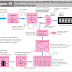Hey, in this article we are going to learn the working principle of a Digital Frequency Meter with its block diagram. A digital frequency meter is a general-purpose measuring device that measures the frequency of an alternating signal or time-varying signal and shows the value in digital format through a display. It is built with different types of electronic components and circuits.

First of all, let's see its block diagram to understand its working principle easily.

The basic principle of the digital voltmeter is it counts the cycles with respect to time and measures the frequency. So, the frequency meter is nothing but a special type of counter. We know that frequency is the no of cycles per second which means 1-hertz frequency the signal completes one cycle per second. Similarly, a 50-hertz frequency means the signal completes 50 cycles per second.

Now, let's understand how this frequency meter works.

As it is a digital meter so all the operation happens in digital form. As you can see in the above diagram, the frequency meter has a Schmitt trigger that converts the analog input signal into digital pulses. Sometimes the input signal needs to be amplified that is why an amplifier can be used before the Schmitt trigger. The Schmitt Trigger converts the analog signal into positive digital pulses in such a way that each pulse is equal to each cycle of the unknown input signal.

For example, if the signal has 50 cycles per second the Schmitt trigger will generate 50 positive pulses per second.

Now, the positive pulses are applied to an AND Gate(it is also known as the Main gate). The output of this main gate is applied to a decimal counter that will count the pulses and show the value of the frequency in the display.

Now, all the fundamental depends upon the main gate. Until we enable the main gate it will not allow passing the pulses from the Schmitt trigger to go to the counter circuit.

Now, we can enable the main gate for a time duration and count the pulses passing through that gate. The no of pulses passing through the gate per time is equal to the frequency of the unknown signal.

For example, we enable the gate for 1 second. And during this 1 second, if 50 pulses passed through the gate, so the frequency of the unknown signal is 50 hertz.

The simple formula is Frequency = No. of Pulses/Time

Now the question is how we enable and disable the main gate in the proper time duration.

As you can see the main gate is basically an AND gate, and it has two input terminals. One terminal is used to receive the pulses from the Schmit trigger. And another terminal can be used to enable or disable the gate. When we apply a high signal, it will enable and when we apply a low signal it will be disabled. For example, to enable the gate for 1 sec we need to provide a high signal for 1 sec.

These timing signals are achieved from the combination of a Crystal Oscillator, Timing Base Control, and gate control flip-flop circuits.

Here, you can see a 1 MHz Crystal Oscillator is used. Here, also a Schmitt Trigger is used at the output of the Crystal Oscillator that converts the analog signal generated from the crystal oscillator into digital signal or pulses.

The frequency divider is a decade frequency divider built with flip flops connected back to back. It is used to divide the frequency by changing the number of pulses per time and duty cycle. For, example if the 50-hertz frequency is divided into 25-hertz frequency, the no of pulses per second will decrease from 50 pulses to 25 pulses per second but the width or duty cycle of the pulses will increase. This width or duty cycle of the pulses will give us time counting.

The time base selector switch is used to select the second so we can change the range of the frequency meter.

The gate control flip flop takes the input signal from the time base selector and generates the output signal to enable or disable the main gate. It also receives the read input pulse to reset the counter or start the counting.

How Digital Frequency Meter (DFM) Works? Learn with DiagramReviewed by Author on December 07, 2022 Rating: 5